# MSBSHSE Solutions For Class 8 Maths Part 1 Chapter 2- Parallel Lines and Transversals

MSBSHSE Solutions For Class 8 Maths Part 1 Chapter 2- Parallel Lines and Transversals are available here. This chapter mainly deals with transversal, angles made by a transversal, corresponding angles, interior angles, alternate angles, properties of angles formed by two parallel lines and transversal, property of corresponding angles, property of alternate angles, property of interior angles. The subject experts at BYJU’S outline the concepts in a clear and precise manner, based on the IQ level of students. Our solution module utilises numerous shortcut tips and practical examples to explain all the exercise questions in a simple and easily understandable language. If you wish to obtain an excellent score, solving Maharashtra Board Solutions for Class 8 is a must.

Here, the students will learn various techniques about the concepts. The solutions to all questions in Maharashtra State Board Class 8 Textbook Part 1 are given here in a detailed and step by step way to help the students understand more effectively. Students can download the solutions of Maharashtra Board from the provided links.

## Download the PDF of Maharashtra Board Solutions For Class 8 Maths Part 1 Chapter 2- Parallel Lines and Transversals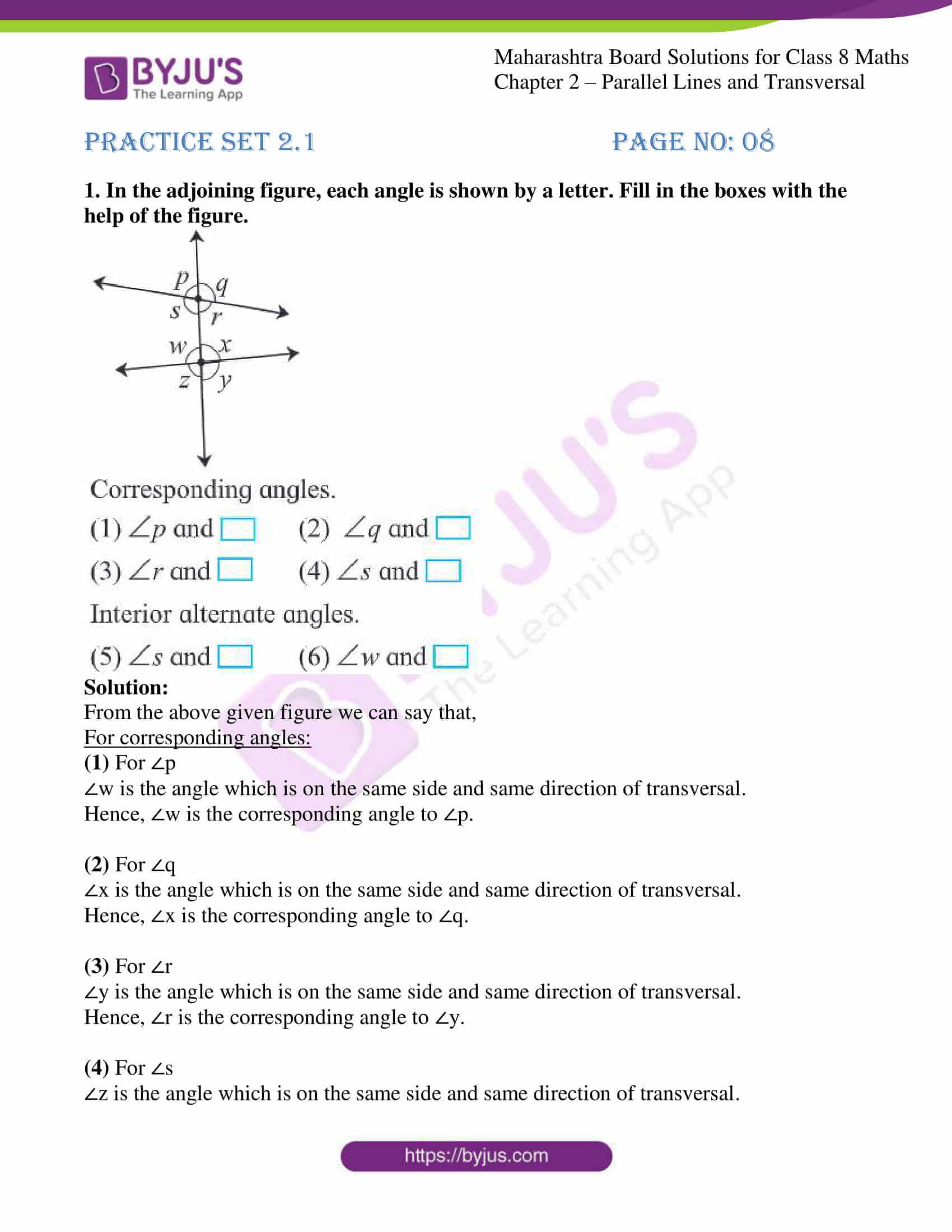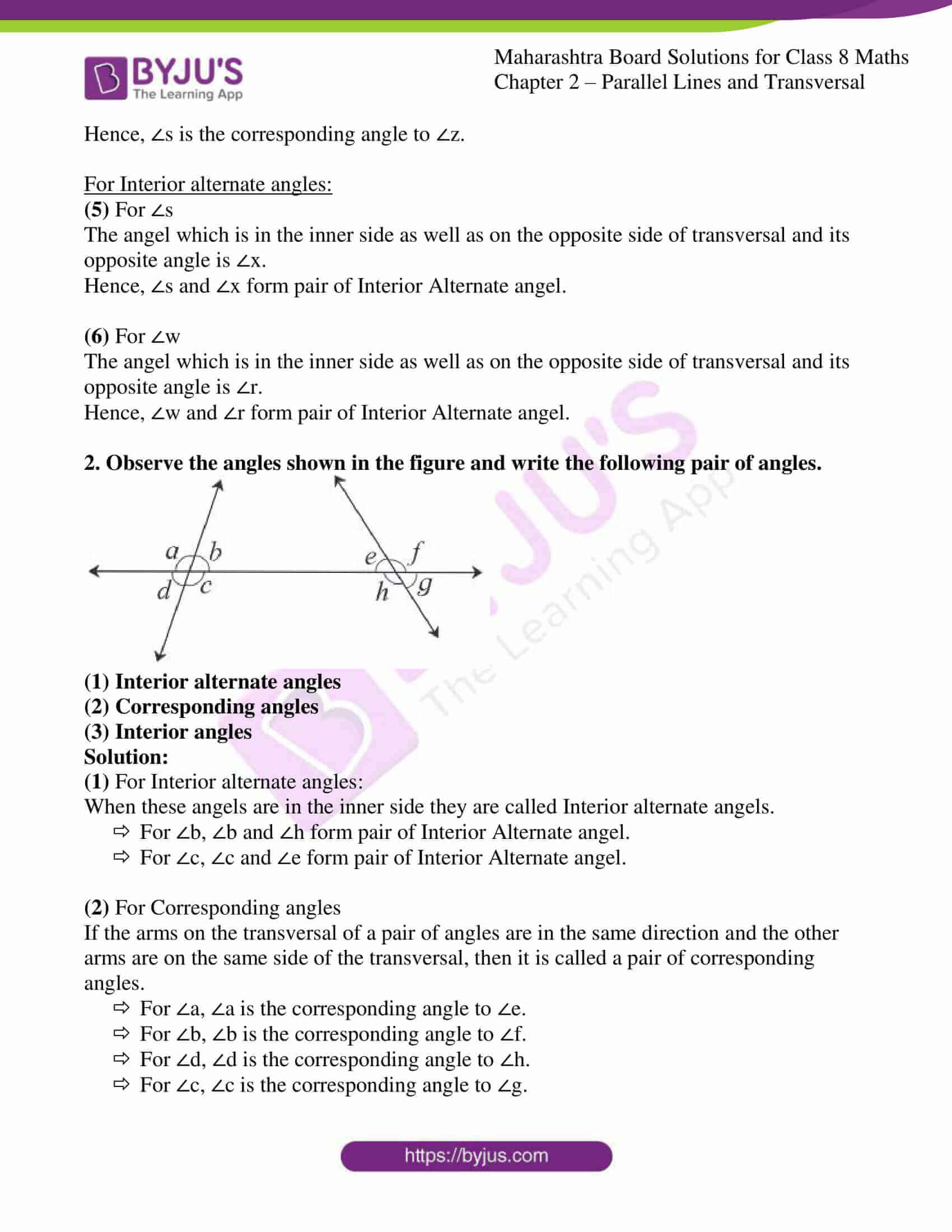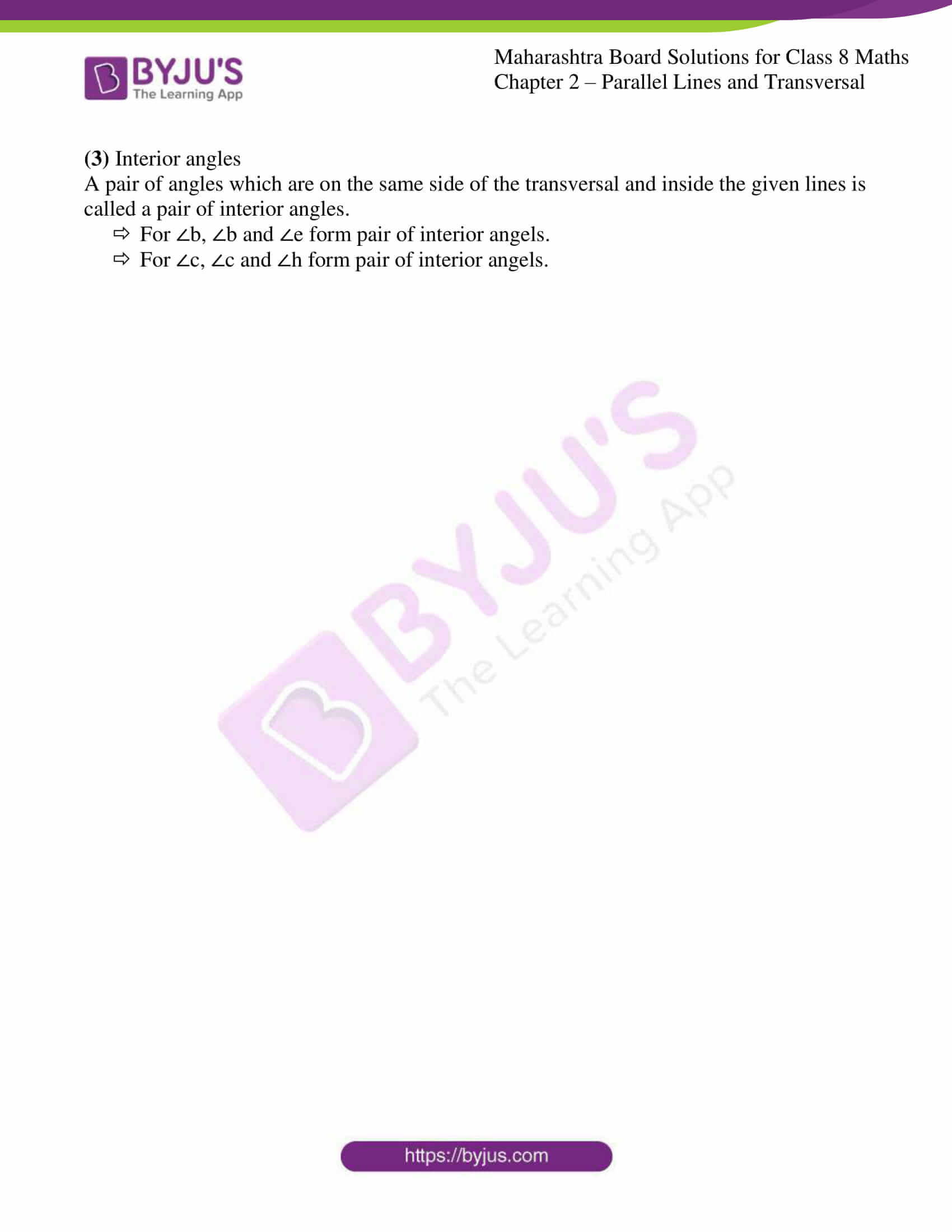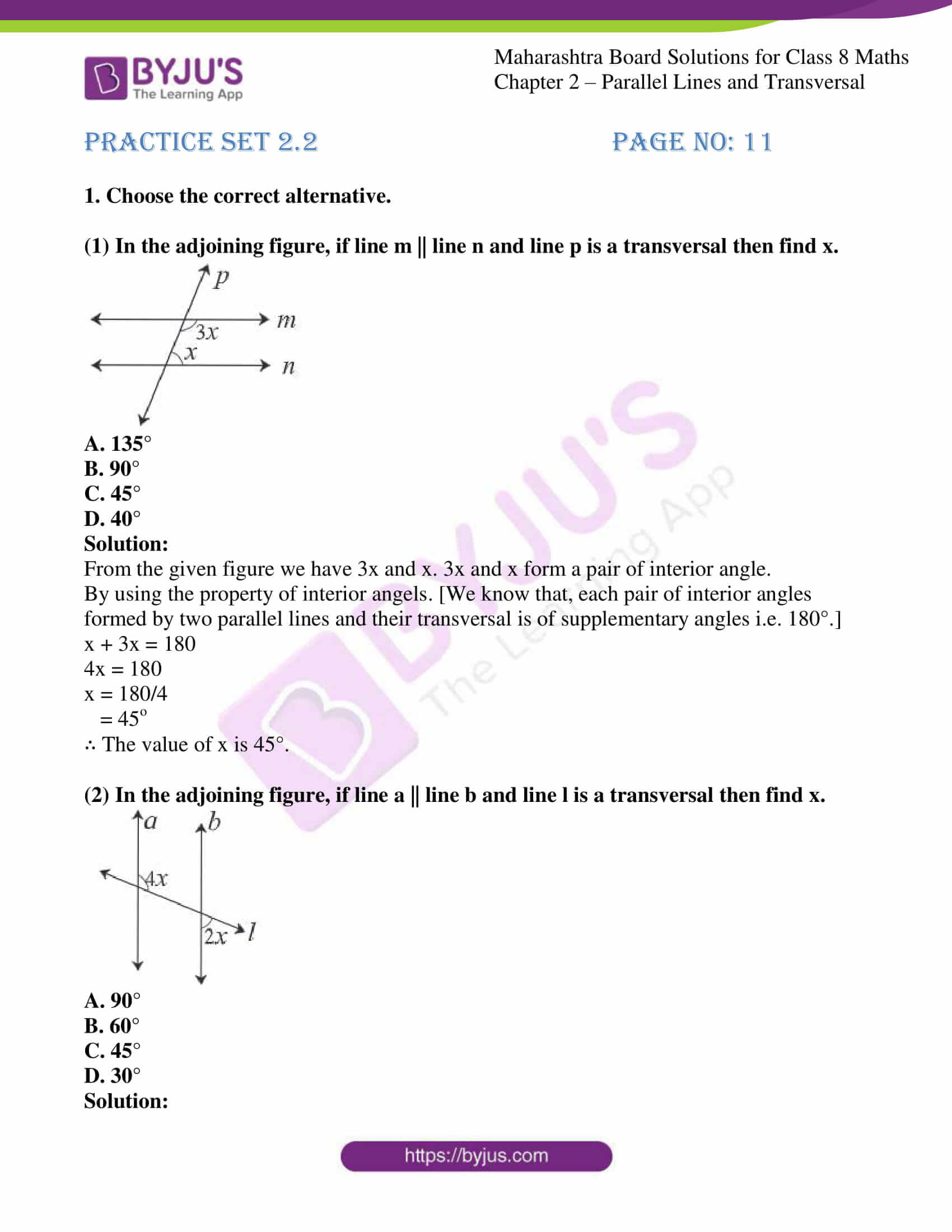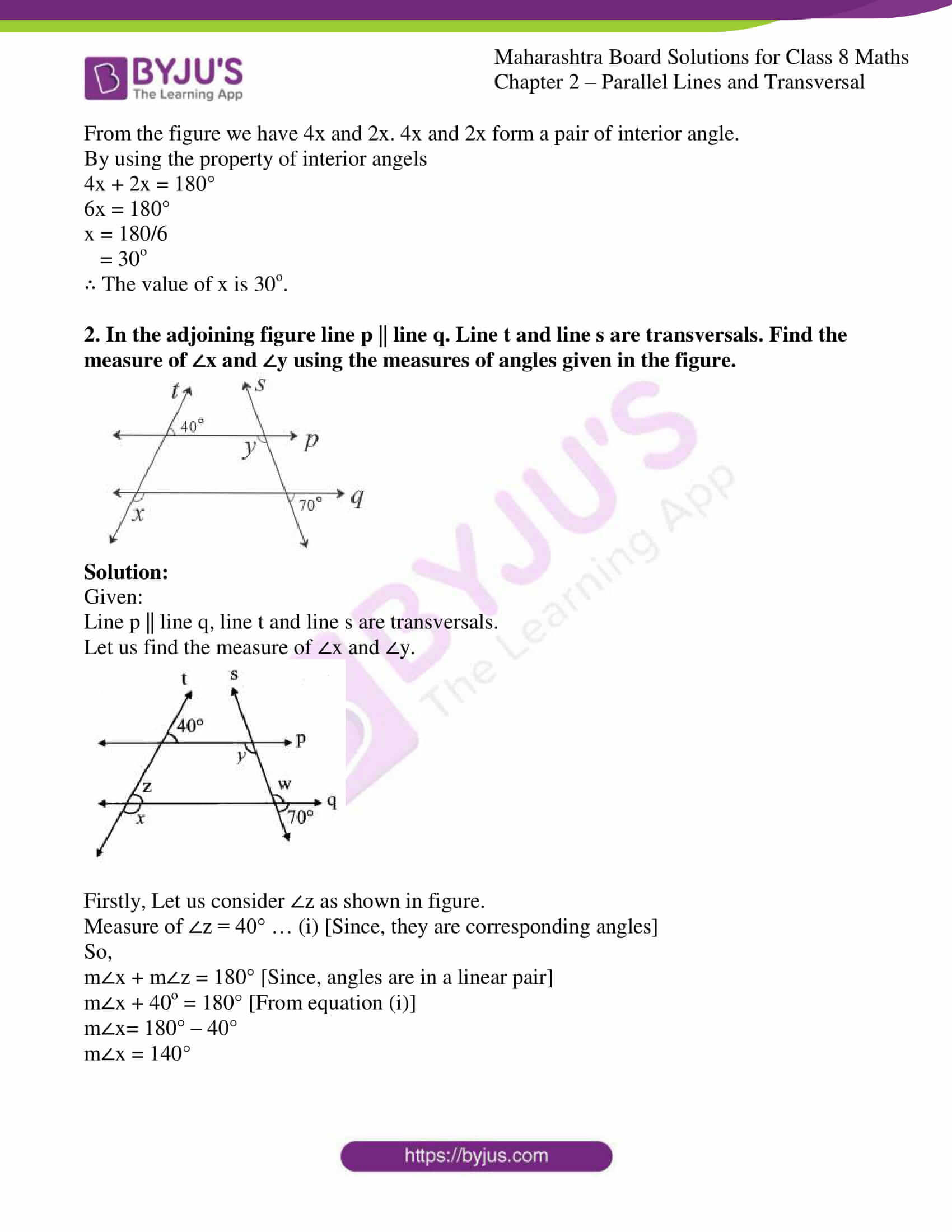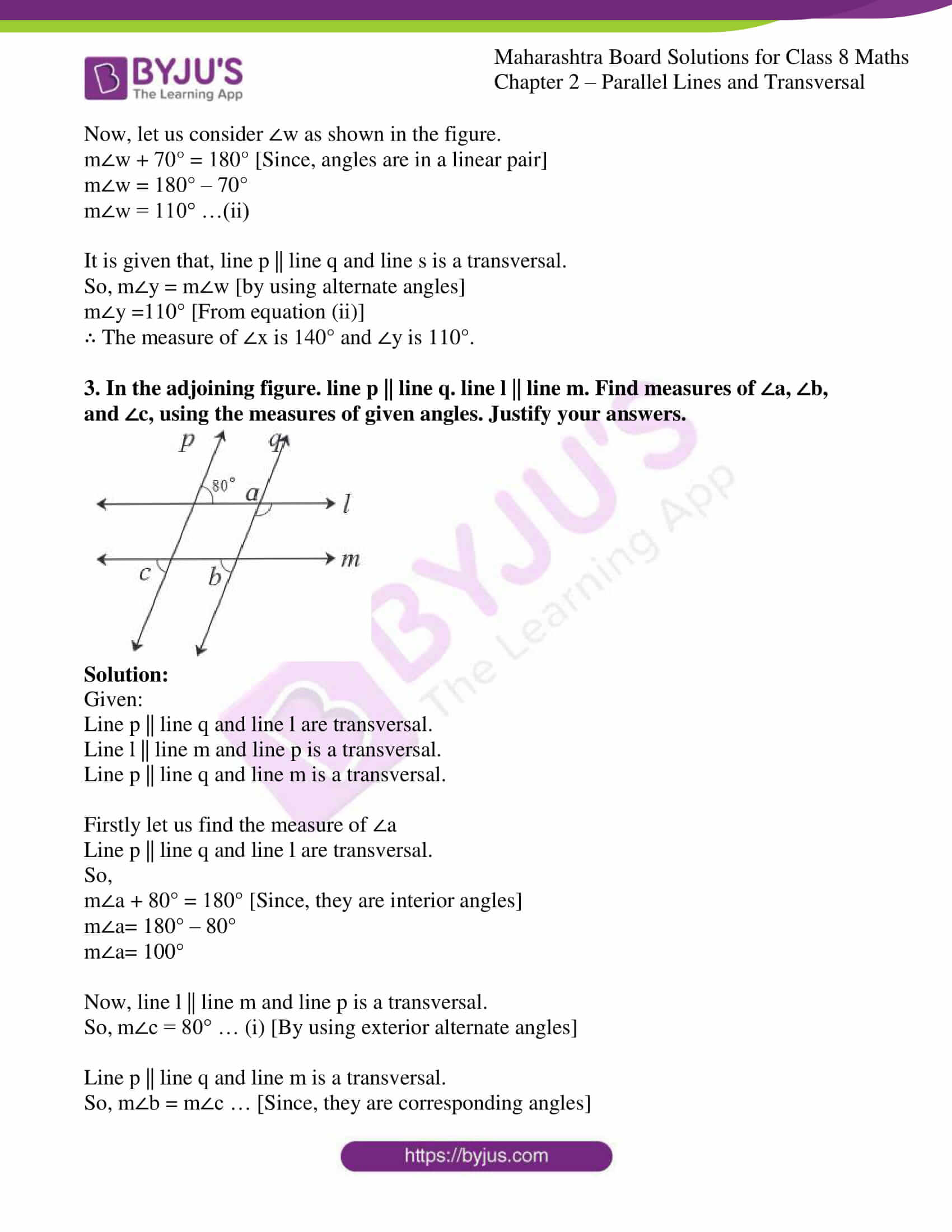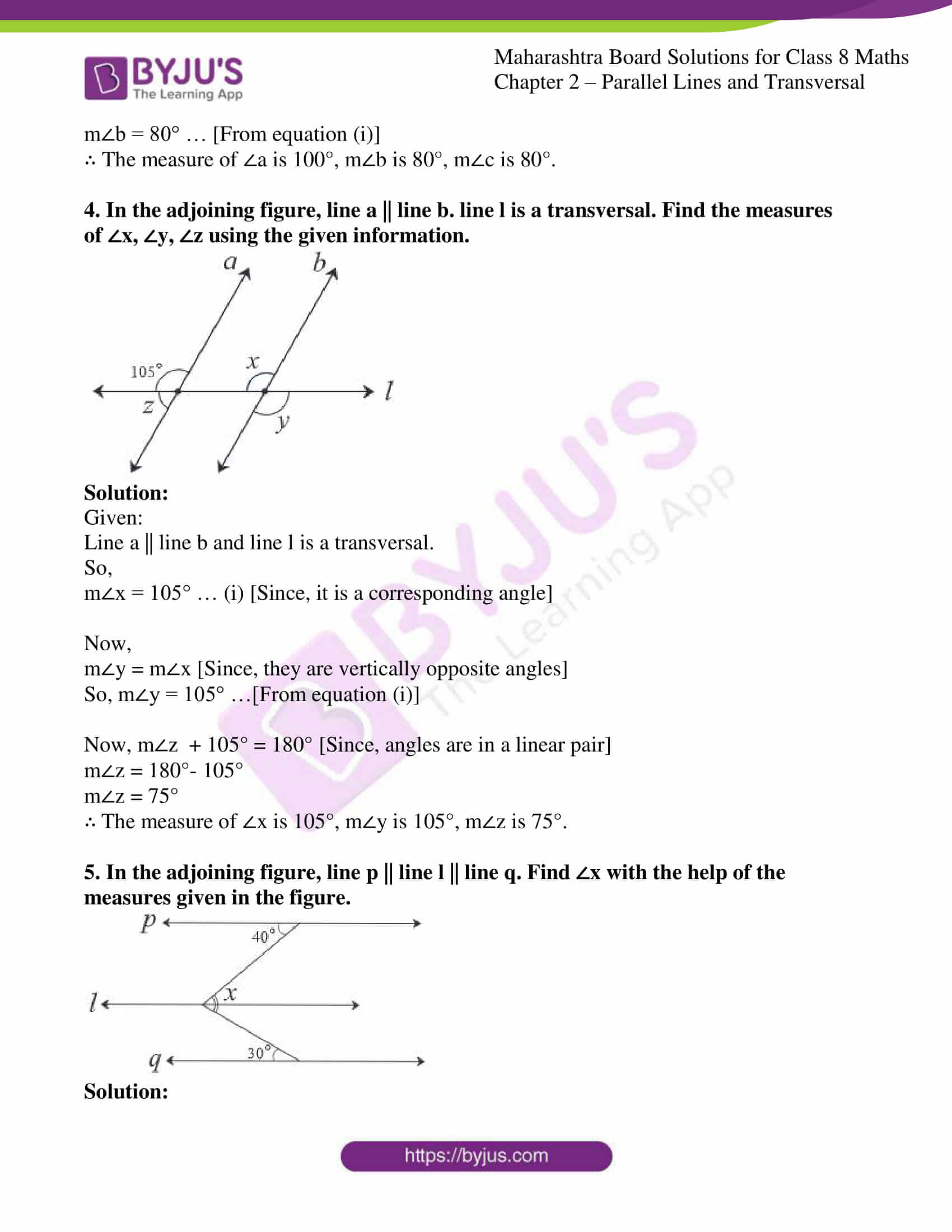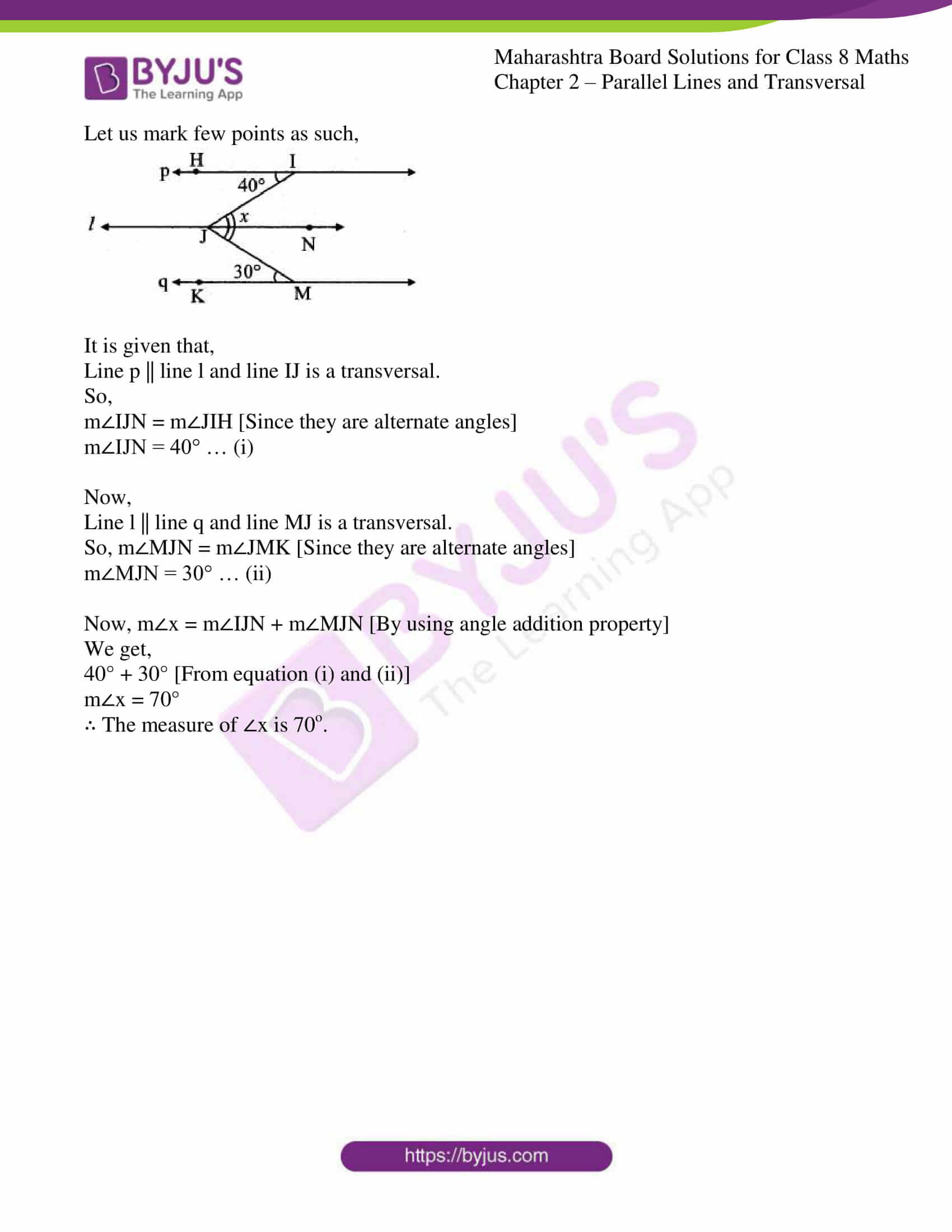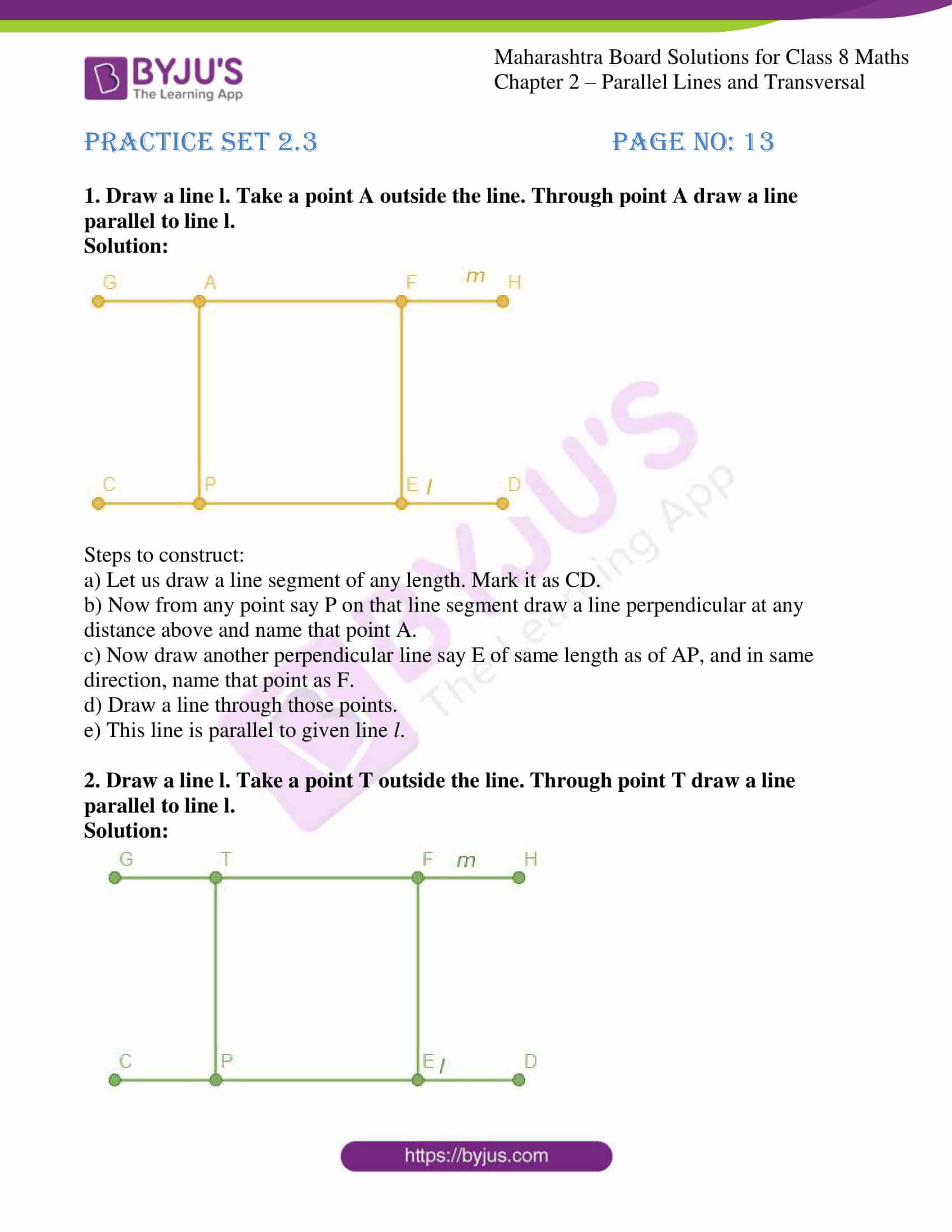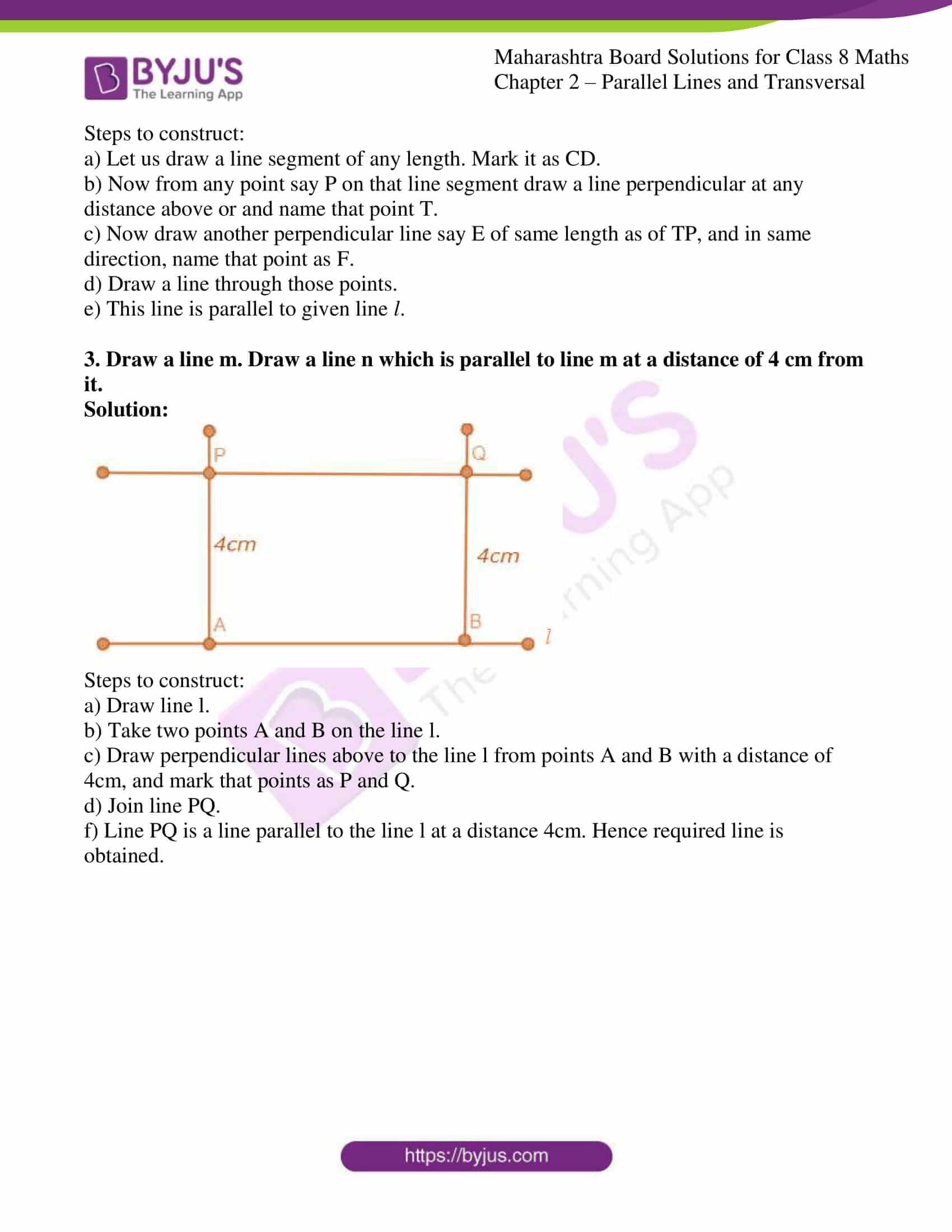### Access answers to Maharashtra Board Solutions For Class 8 Maths Part 1 Chapter 2- Parallel Lines and Transversals

Practice set 2.1 PAGE NO: 08

1. In the adjoining figure, each angle is shown by a letter. Fill in the boxes with the help of the figure.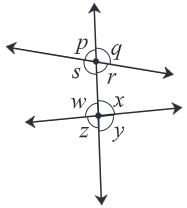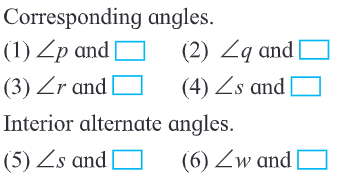Solution:

From the above given figure we can say that,

For corresponding angles:

(1) For ∠p

∠w is the angle which is on the same side and same direction of transversal.

Hence, ∠w is the corresponding angle to ∠p.

(2) For ∠q

∠x is the angle which is on the same side and same direction of transversal.

Hence, ∠x is the corresponding angle to ∠q.

(3) For ∠r

∠y is the angle which is on the same side and same direction of transversal.

Hence, ∠r is the corresponding angle to ∠y.

(4) For ∠s

∠z is the angle which is on the same side and same direction of transversal.

Hence, ∠s is the corresponding angle to ∠z.

For Interior alternate angles:

(5) For ∠s

The angel which is in the inner side as well as on the opposite side of transversal and its opposite angle is ∠x.

Hence, ∠s and ∠x form pair of Interior Alternate angel.

(6) For ∠w

The angel which is in the inner side as well as on the opposite side of transversal and its opposite angle is ∠r.

Hence, ∠w and ∠r form pair of Interior Alternate angel.

2. Observe the angles shown in the figure and write the following pair of angles.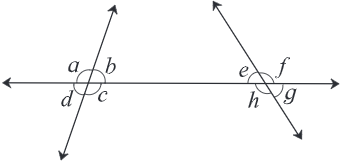(1) Interior alternate angles
(2) Corresponding angles
(3) Interior angles

Solution:

(1) For Interior alternate angles:

When these angels are in the inner side they are called Interior alternate angels.

• For ∠b, ∠b and ∠h form pair of Interior Alternate angel.
• For ∠c, ∠c and ∠e form pair of Interior Alternate angel.

(2) For Corresponding angles

If the arms on the transversal of a pair of angles are in the same direction and the other arms are on the same side of the transversal, then it is called a pair of corresponding angles.

• For ∠a, ∠a is the corresponding angle to ∠e.
• For ∠b, ∠b is the corresponding angle to ∠f.
• For ∠d, ∠d is the corresponding angle to ∠h.
• For ∠c, ∠c is the corresponding angle to ∠g.

(3) Interior angles

A pair of angles which are on the same side of the transversal and inside the given lines is called a pair of interior angles.

• For ∠b, ∠b and ∠e form pair of interior angels.
• For ∠c, ∠c and ∠h form pair of interior angels.

Practice set 2.2 PAGE NO: 11

1. Choose the correct alternative.

(1) In the adjoining figure, if line m || line n and line p is a transversal then find x.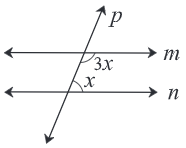A. 135°
B. 90°
C. 45°
D. 40°

Solution:

From the given figure we have 3x and x. 3x and x form a pair of interior angle.

By using the property of interior angels. [We know that, each pair of interior angles formed by two parallel lines and their transversal is of supplementary angles i.e. 180°.]

x + 3x = 180

4x = 180

x = 180/4

= 45o

∴ The value of x is 45°.

(2) In the adjoining figure, if line a || line b and line l is a transversal then find x.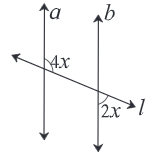A. 90°
B. 60°
C. 45°
D. 30°

Solution:

From the figure we have 4x and 2x. 4x and 2x form a pair of interior angle.

By using the property of interior angels

4x + 2x = 180°

6x = 180°

x = 180/6

= 30o

∴ The value of x is 30o.

2. In the adjoining figure line p || line q. Line t and line s are transversals. Find the measure of ∠x and ∠y using the measures of angles given in the figure.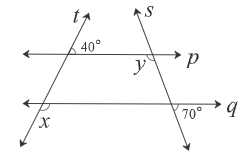Solution:

Given:

Line p || line q, line t and line s are transversals.

Let us find the measure of ∠x and ∠y.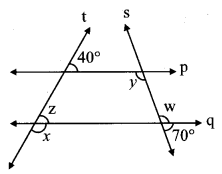Firstly, Let us consider ∠z as shown in figure.

Measure of ∠z = 40° … (i) [Since, they are corresponding angles]

So,

m∠x + m∠z = 180° [Since, angles are in a linear pair]

m∠x + 40o = 180° [From equation (i)]

m∠x= 180° – 40°

m∠x = 140°

Now, let us consider ∠w as shown in the figure.

m∠w + 70° = 180° [Since, angles are in a linear pair]

m∠w = 180° – 70°

m∠w = 110° …(ii)

It is given that, line p || line q and line s is a transversal.

So, m∠y = m∠w [by using alternate angles]

m∠y =110° [From equation (ii)]

∴ The measure of ∠x is 140° and ∠y is 110°.

3. In the adjoining figure. line p || line q. line l || line m. Find measures of ∠a, ∠b, and ∠c, using the measures of given angles. Justify your answers.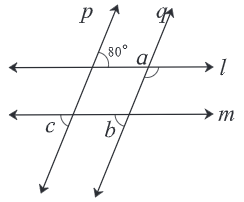Solution:

Given:

Line p || line q and line l are transversal.

Line l || line m and line p is a transversal.

Line p || line q and line m is a transversal.

Firstly let us find the measure of ∠a

Line p || line q and line l are transversal.

So,

m∠a + 80° = 180° [Since, they are interior angles]

m∠a= 180° – 80°

m∠a= 100°

Now, line l || line m and line p is a transversal.

So, m∠c = 80° … (i) [By using exterior alternate angles]

Line p || line q and line m is a transversal.

So, m∠b = m∠c … [Since, they are corresponding angles]

m∠b = 80° … [From equation (i)]

∴ The measure of ∠a is 100°, m∠b is 80°, m∠c is 80°.

4. In the adjoining figure, line a || line b. line l is a transversal. Find the measures of ∠x, ∠y, ∠z using the given information.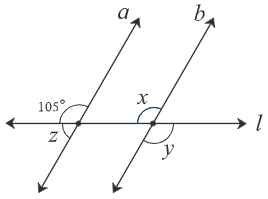Solution:

Given:

Line a || line b and line l is a transversal.

So,

m∠x = 105° … (i) [Since, it is a corresponding angle]

Now,

m∠y = m∠x [Since, they are vertically opposite angles]

So, m∠y = 105° …[From equation (i)]

Now, m∠z + 105° = 180° [Since, angles are in a linear pair]

m∠z = 180°- 105°

m∠z = 75°

∴ The measure of ∠x is 105°, m∠y is 105°, m∠z is 75°.

5. In the adjoining figure, line p || line l || line q. Find ∠x with the help of the measures given in the figure.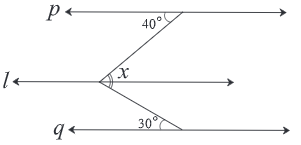Solution:

Let us mark few points as such,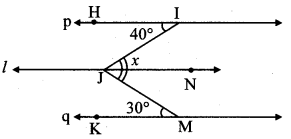It is given that,

Line p || line l and line IJ is a transversal.

So,

m∠IJN = m∠JIH [Since they are alternate angles]

m∠IJN = 40° … (i)

Now,

Line l || line q and line MJ is a transversal.

So, m∠MJN = m∠JMK [Since they are alternate angles]

m∠MJN = 30° … (ii)

Now, m∠x = m∠IJN + m∠MJN [By using angle addition property]

We get,

40° + 30° [From equation (i) and (ii)]

m∠x = 70°

∴ The measure of ∠x is 70o.

Practice set 2.3 PAGE NO: 13

1. Draw a line l. Take a point A outside the line. Through point A draw a line parallel to line l.

Solution: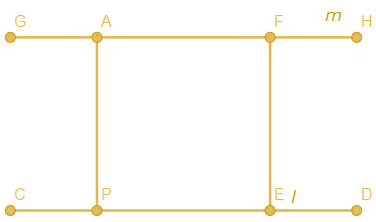Steps to construct:

a) Let us draw a line segment of any length. Mark it as CD.

b) Now from any point say P on that line segment draw a line perpendicular at any distance above and name that point A.

c) Now draw another perpendicular line say E of same length as of AP, and in same direction, name that point as F.

d) Draw a line through those points.

e) This line is parallel to given line l.

2. Draw a line l. Take a point T outside the line. Through point T draw a line parallel to line l.

Solution: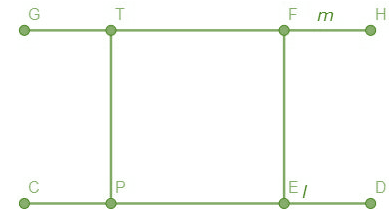Steps to construct:

a) Let us draw a line segment of any length. Mark it as CD.

b) Now from any point say P on that line segment draw a line perpendicular at any distance above or and name that point T.

c) Now draw another perpendicular line say E of same length as of TP, and in same direction, name that point as F.

d) Draw a line through those points.

e) This line is parallel to given line l.

3. Draw a line m. Draw a line n which is parallel to line m at a distance of 4 cm from it.

Solution: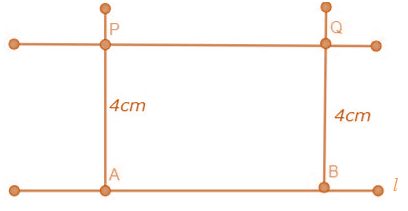Steps to construct:

a) Draw line l.

b) Take two points A and B on the line l.

c) Draw perpendicular lines above to the line l from points A and B with a distance of 4cm, and mark that points as P and Q.

d) Join line PQ.

f) Line PQ is a line parallel to the line l at a distance 4cm. Hence, the required line is obtained.

The lines in the same plane, which do not intersect each other are called parallel lines. If a line intersects two lines in two distinct points, then that line is called transversal of those two lines. To get to know more about the concepts covered in this chapter, students can refer to Parallel Lines and Transversals at BYJU’S.

## Frequently Asked Questions on Maharashtra State Board Solutions for Class 8 Maths Chapter 2 Parallel Lines and Transversals

### Will I have to make any payment to access these Maharashtra State Board Solutions for Class 8 Maths  Chapter 2 Parallel Lines and Transversals?

No, there is no need to pay any money. We have made these solutions available online free for download. Students can access them from our site by entering the login details. Or else, the scrollable PDF is also accessible. Those who wish to can also check out the questions and the solutions from our webpage.

### Are these Maharashtra State Board Solutions for Class 8 Maths Chapter 2 Parallel Lines and Transversals helpful?

Yes. These solutions are useful and they set the basis for questions that could get asked in the board exams. Students are also advised to practise these questions first and then refer back to the solutions to analyse their performance and then rectify the mistakes, so that they can avoid making any during the board exams.

### Is Maharashtra Board Class 8 Maths Solutions of Chapter 2 difficult?

No, Maharashtra Board Class 9 Maths Solutions of Chapter 2  is not half as difficult as it seems. You can easily understand concepts of the chapter if you refer to this chapterwise solutions . You can also go through your syllabus course structure and unit-wise weightage thoroughly to know how much weightage the chapter will carry for the final exam.